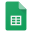# Torque - Rotatational Equilibrium

Plan an investigation to provide evidence that the change in an object’s motion depends on the sum of the forces on the object and the mass of the object. [Clarification Statement: Emphasis is on balanced (Newton’s First Law) and unbalanced forces in a system, qualitative comparisons of forces, mass and changes in motion (Newton’s Second Law), frame of reference, and specification of units.] [Assessment Boundary: Assessment is limited to forces and changes in motion in one-dimension in an inertial reference frame and to change in one variable at a time. Assessment does not include the use of trigonometry.]

## Warm-up Questions - Answer in the Quickwrite

• What is a lever?
• What is torque?
• What are examples in the body of first, second, and third class levers?## Activity

• Balance the meter stick at the 0.50m (50 cm) mark.
• Measure the mass of two hanger/block combinations, and determine the force (weight) of each in newtons by multiplying by the acceleration due to gravity (F=mg)
• Place one on the right side of the fulcrum, and the other on the left side so that the meter stick balances as shown in the diagram
• Put your values in the table below.Torque

Open torque worksheet

## Questions

Use the quickwrite to answer the following questions:

1. Examine the graph that displays class data of the relationship between clockwise and counter-clockwise torque in a balanced system. Describe the relationship between clockwise torque and counter-clockwise torque in a balanced system. Write an equation that describes this relationship.
2. Mechanical advantage is a measure of the force amplification achieved by using a tool, mechanical device or machine system. What is the formula for mechanical advantage?
3. What is sacrificed when the mechanical advantage is greater than 1? What is gained?
4. Explain the following diagram